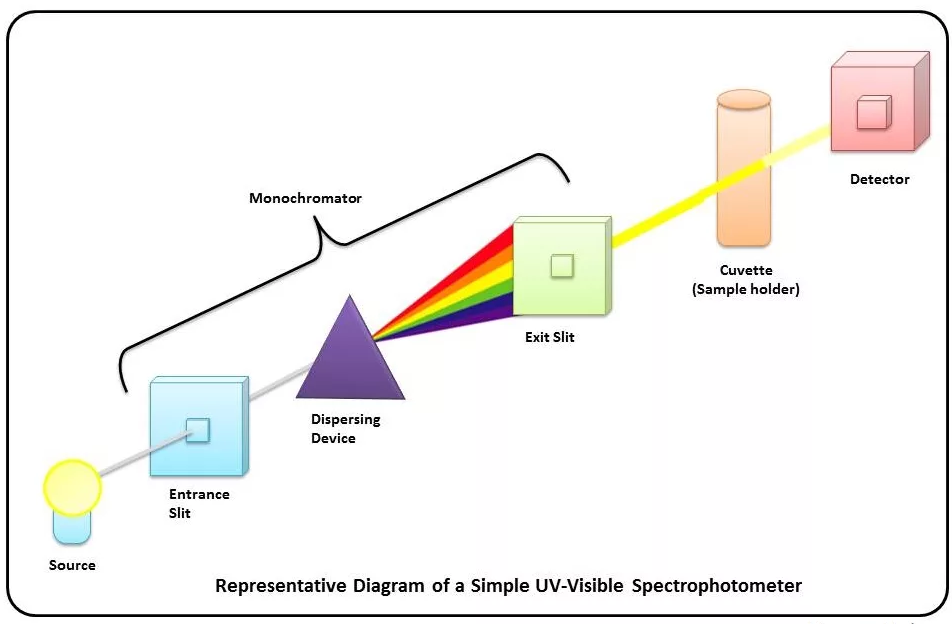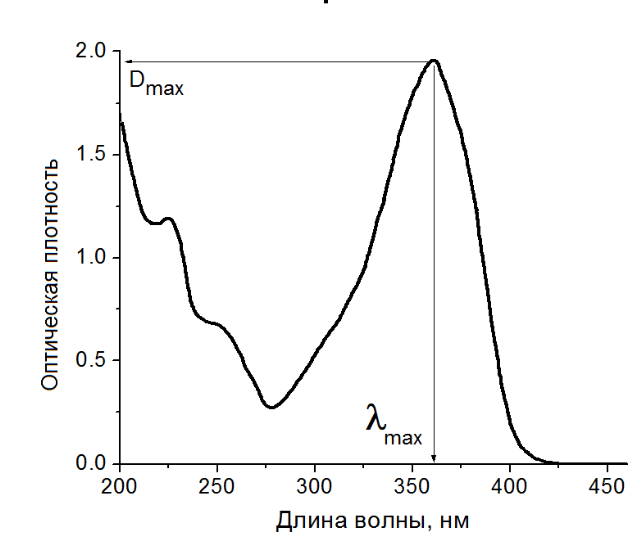# Quantitative method of water quality detection by spectrophotometry

At present, the rapid detection of water quality mostly adopts spectrophotometry, which is an analysis method established based on the selective absorption of light by the measured substance. It is mainly used to detect the content of trace components in water quality samples, such as ammonia nitrogen and residual in drinking water. Chlorine, heavy metals, volatile phenols, total phosphorus and other substances. Compared with the traditional chemical analysis method, it has the characteristics of high sensitivity, high accuracy, simple and quick operation and wide application range. But if you want to ensure the accuracy of spectrophotometry, you must know its quantitative method.## Quantitative method of water quality detection by spectrophotometry

### 1. Standard curve method

The standard curve method is based on the law of light absorption. If the thickness of the liquid layer and the wavelength of the incident light remain unchanged, within a certain concentration range, the measured absorbance is proportional to the concentration of the substance to be tested in the solution. First prepare a series of standard solutions of known and accurate concentration, measure the absorbance A at the selected wavelength, and then use the concentration c of the standard solution as the abscissa and the corresponding absorbance A as the ordinate to draw the Ac relationship diagram, and get a The straight line passing through the origin of the coordinates is called the standard curve. The absorbance of the sample solution is measured under the same conditions, and the concentration of the sample solution can be found from the standard curve.### 2. Comparative Law

Under the same conditions, prepare the test sample solution and a standard solution with a similar concentration, and measure the absorbance of the standard solution and the absorbance of the sample solution at the selected wavelength. According to Lambert Beer's law:

A=Kbc

A standard=Kbc standard

A-like=Kbc-like

c sample=c standard A sample/A standard

It is more suitable for the situation where the linear relationship is good and the origin is passed

### 3. Unary linear regression method

Use absorbance A and concentration c to make the work curve, the abscissa is the independent variable c, the ordinate is the dependent variable A, usually the independent variable can be controlled and accurately measured, and the dependent variable is a random variable with errors. Draw a straight line according to the scattered points, call this straight line a regression line

If a straight line passes through all experimental points, it can be said that A and c are closely linearly related. If the experimental points are not completely on a straight line, in order to minimize the error, further linear regression performance is required, and the regression equation is

Y=a+bx

Where a--the intercept of the regression line on the vertical axis;

b--The slope of the regression line;

Determine the two parameters a and b to get the linear regression equation. The two parameters a and b can be obtained by the least square method in mathematics. A calculator with linear regression can achieve this calculation, just enter the concentration of the standard series and the corresponding absorbance.

After establishing the linear regression equation, substituting the measured absorbance of the sample into the equation, the concentration of the sample can be calculated.